# TwoSlabDiffusion

Model number
0212

Diffusion through two adjoining slabs with different diffusion coefficients.

## Description

Two slabs with different diffusion coefficients are placed next to each other. They are insulated along their lengths, but exposed at their ends. The discontinuity in diffusion coefficients where the slabs meet is modeled by a continuous function over a single spatial step. It is essential that the functional equation for the discontinuous diffusion coefficient be represented by a continuous function for the partial differential equation solvers. Two approaches are given: 1.) The diffusion is given as a piecewise continuous function joining the two different diffusion coefficients and linearly changing value from D1 to D2 from b-x.delta/2 to b+x.delta/2. 2.) The diffusion is given by a smooth hyperbolic function. The analytic steady state solution is from the problem where the diffusion coefficients form a discontinuous function. The solution for the Steady State Problem is derived from CanalyticSS(x) = if (x<=b) C1*x+C2 else C3*x+C4, where the coefficients C1, C2, C3, and C4 satisfy at x=x.min: C1*x.min + C2 = Cleft at x=b: C1*b + C2 = C3*b +C4 (concentration is continuous at x=b) D1*C1 = D2*C3 (flux on both sides of x=b are in equilibrium) at x=x.max: C3*x.max + C4 = Cright. See Notes for Figure description

## Equations

#### Two separate partial differential equation models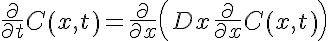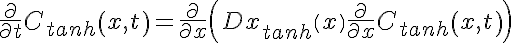#### where the diffusion coefficients as a function of x are given respectively by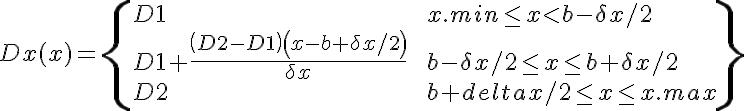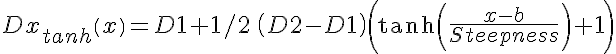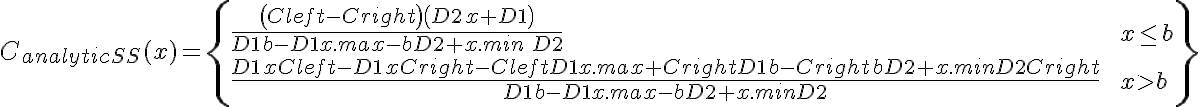The equations for this model may be viewed by running the JSim model applet and clicking on the Source tab at the bottom left of JSim's Run Time graphical user interface. The equations are written in JSim's Mathematical Modeling Language (MML). See the Introduction to MML and the MML Reference Manual. Additional documentation for MML can be found by using the search option at the Physiome home page.

References

None.

Key terms
Diffusion
slab
two slabs
PDE
diffusion profiles
delay
fluxes
Acknowledgements

Please cite https://www.imagwiki.nibib.nih.gov/physiome in any publication for which this software is used and send one reprint to the address given below:
The National Simulation Resource, Director J. B. Bassingthwaighte, Department of Bioengineering, University of Washington, Seattle WA 98195-5061.

Model development and archiving support at https://www.imagwiki.nibib.nih.gov/physiome provided by the following grants: NIH U01HL122199 Analyzing the Cardiac Power Grid, 09/15/2015 - 05/31/2020, NIH/NIBIB BE08407 Software Integration, JSim and SBW 6/1/09-5/31/13; NIH/NHLBI T15 HL88516-01 Modeling for Heart, Lung and Blood: From Cell to Organ, 4/1/07-3/31/11; NSF BES-0506477 Adaptive Multi-Scale Model Simulation, 8/15/05-7/31/08; NIH/NHLBI R01 HL073598 Core 3: 3D Imaging and Computer Modeling of the Respiratory Tract, 9/1/04-8/31/09; as well as prior support from NIH/NCRR P41 RR01243 Simulation Resource in Circulatory Mass Transport and Exchange, 12/1/1980-11/30/01 and NIH/NIBIB R01 EB001973 JSim: A Simulation Analysis Platform, 3/1/02-2/28/07.